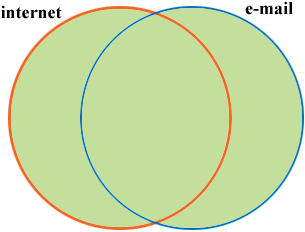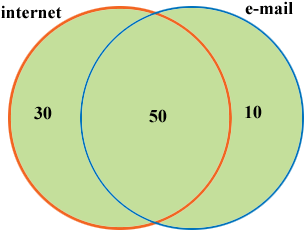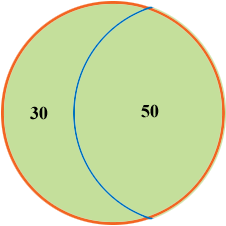SEARCH HOMEMath Central Quandaries & QueriesQuestion from Sandy: Hi I am a TA and up until this year worked with elementary students so I'm really out of my element trying to help grade 12 students. Can you be so kind as to show me the easiest steps involved in solving this problem. I actually found it in the archives but it was in regards to a Venn Diagram and I became confused. Is there an easier way which can be shown to students who are really struggling with this unit TI-83 calculators are allowed? The problem is as follows- In a certain school, it is known that 80% of the students use the internet for school projects, 60% use e-mail on a regular basis, and 90% use the internet for school projects or for e-mail on a regular basis. A student from this school is selected at random Determine the probability that the student used e-mail, given that the student used the internet for school projects. Thank you for your help and hopefully I can get a step ahead of the students during spring break. Sincerely, SandyHi Sandy,

In the problem you mention the student is instructed to use a diagram so let's do it that way. Here is how I would start.I am going to assume there are 100 students in the class so I can deal with counts rather than percentages. You are told there are 80 students in the orange circle, 60 students in the blue circle but all together there are only 90 students who use either the internet or email. 80 + 60 = 140 and 140 - 90 = 50 so 50 of the students got counted twice. These are the students in the overlap of the two circles so now you can see how the 90 students are arranged, 80 students in the internet circle, 60 in the e-mail circle and 90 students in total.The question asked is "Determine the probability that the student used e-mail, given that the student used the internet for school projects." The clause "given that the student used the internet for school projects" tells you that you need to focus on the internet circle.There are 80 students in this circle and you want the probability that if you select one of them then the student you select also uses e-mail. 50 of the students in this circle use e-mail so the probability is 50/80 = 5/8.

I like this solution since the diagrams really help me see what is going on. You can however solve the problem just using formulas. If E represents the students who use e-mail and I represents the students who use the internet then

P(I or E) = P(I) + P(E) - P(I and E).

You know P(I or E) = 0.90, P(I) = 0.80 and P(E) = 0.60 so

0.90 = 0.80 + 0.60 - P(I and E)

so

P(I and E) = 0.80 + 0.60 - 0.90 = 0.50.

The probability that the student used e-mail, given that the student used the internet for school projects is

P(E|I) = P(I and E)/P(I) = 0.50/0.80 = 5/8.

If this were my class I would show the students both techniques.

I hope this helps,
PennyMath Central is supported by the University of Regina and The Pacific Institute for the Mathematical Sciences.